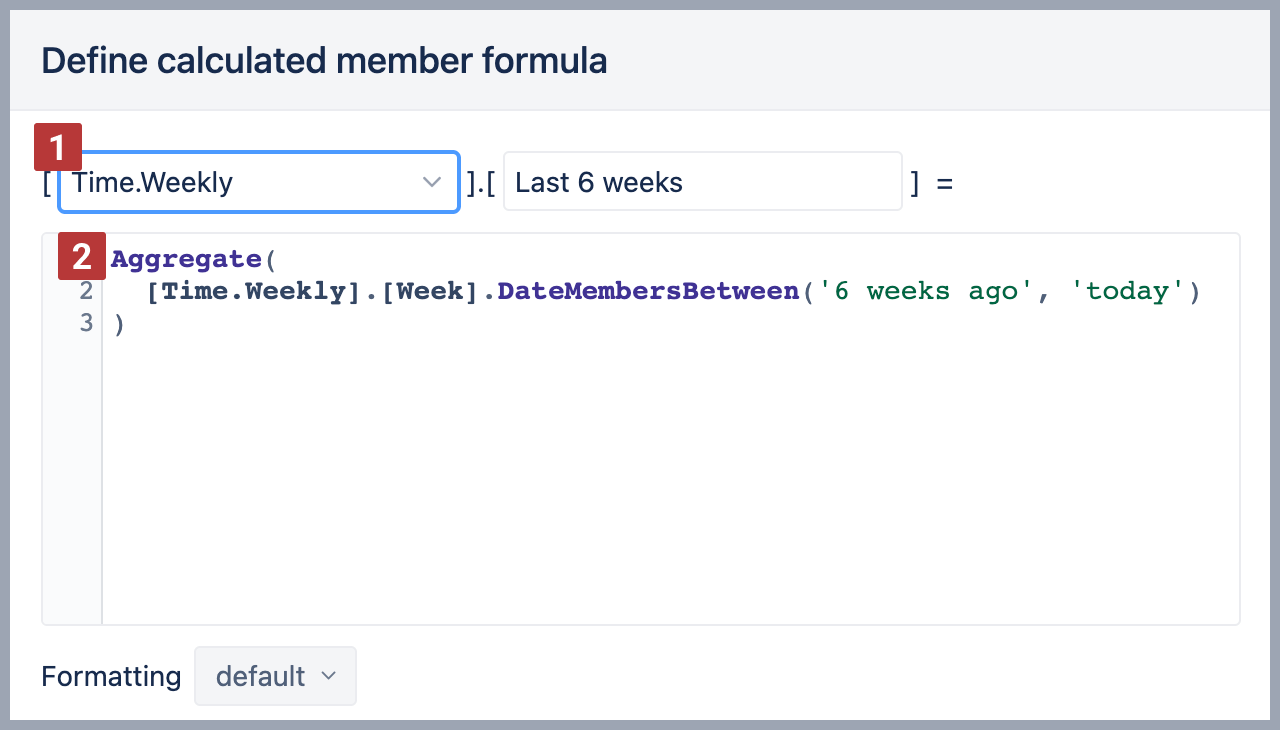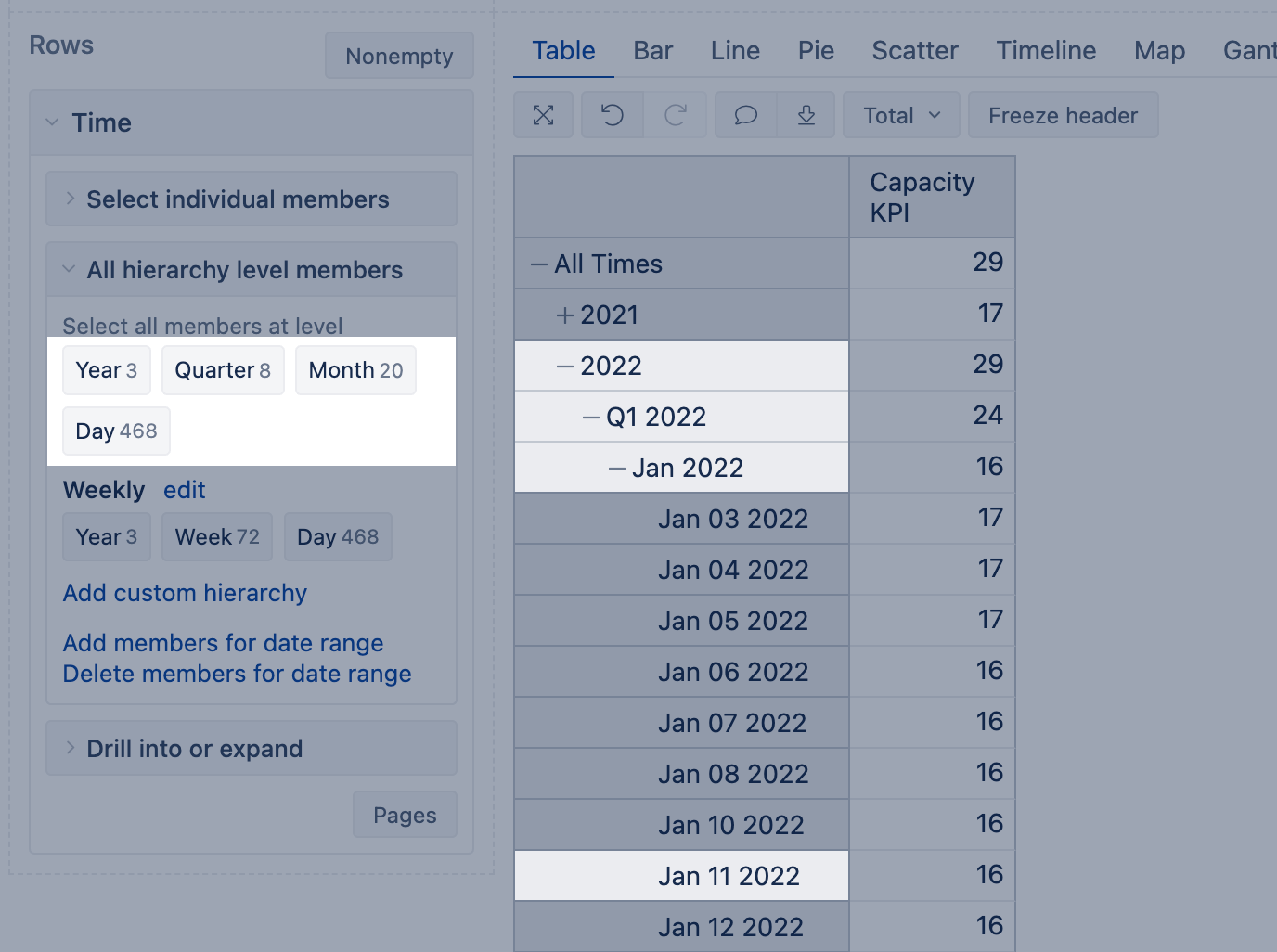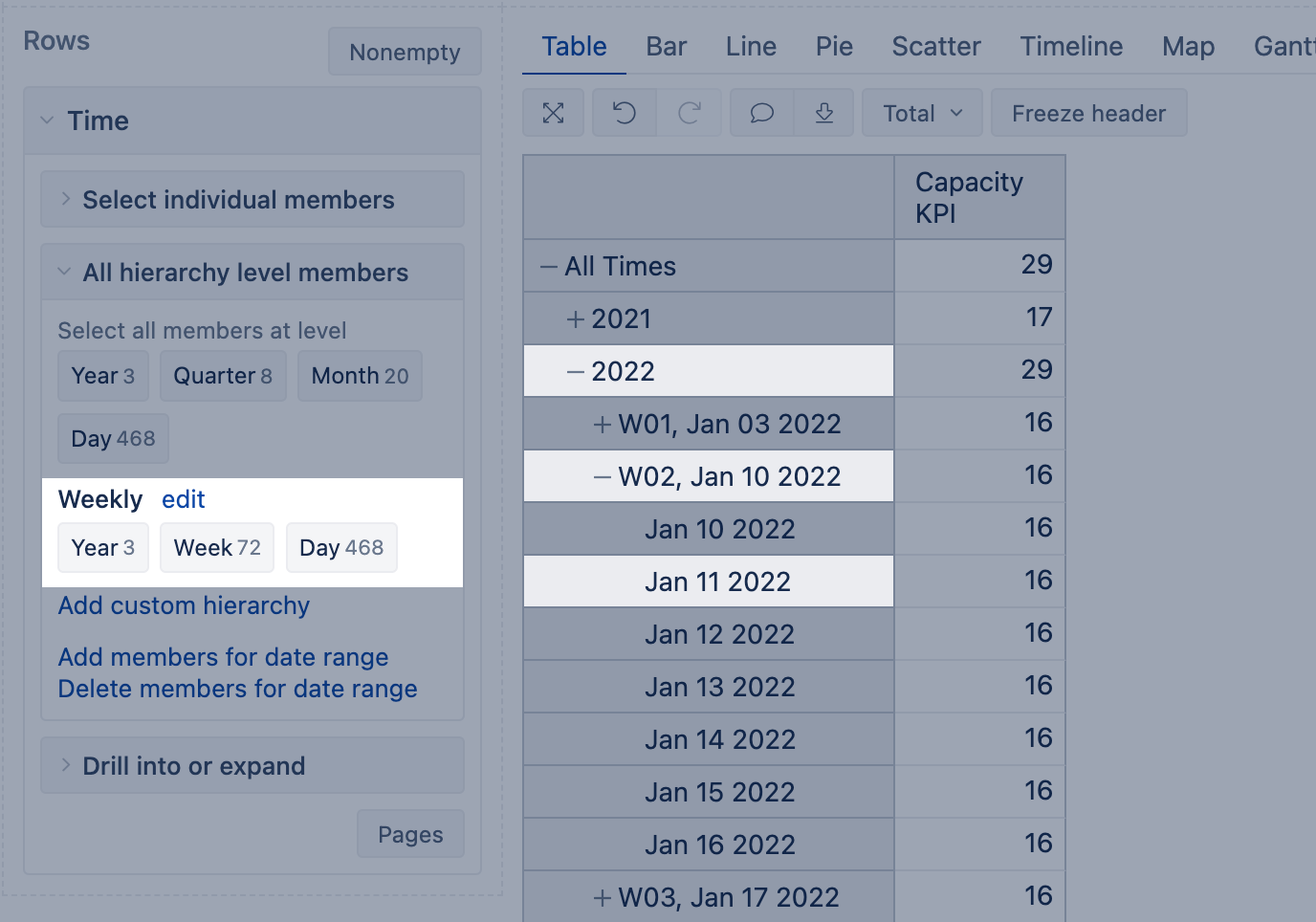All eazyBI for Jira eazyBI for Confluence Private eazyBI

# Calculated members in Time dimension eazyBI.com eazyBI for Confluence eazyBI for Jira Private eazyBI

Time is an important dimension in eazyBI; it allows to group and filter data by different date fields. In eazyBI is one Time dimension, and combined with a measure, it groups data by a date related to that measure. See the documentation on Time dimension for more details on how to use the Time dimension in a report.

You can filter the report by specific time periods - either imported periods (specific date, like Aug 31, 2022, or month like Jan 2022) or custom periods (for example, a period from Mar 5, 2022, to Aug 22, 2022, or a current year or last three week ) created as calculated members in Time dimension.

After you have defined calculated members in the Time dimension, they can be used on report pages as filters and addressed in calculated measures.

## Ground rules for calculated members in Time

There are some ground rules when creating calculated members in the Time dimension:

• Remember that calculated member is a member representing a time period, not a date (i.e. not a value with a date data type), even if the calculated member contains only one day member, like today or yesterday.
• When creating a calculated member in a Time dimension, use only members from Time dimension; those members must be from the same hierarchy. Don't mix dimensions and hierarchies in calculated members!
• Always save the member to the Time hierarchy, which is used in the formula. In other words, on saving the member, ensure the referred Time dimension members match the hierarchy where the calculated member is created . You will be warned if not, do not ignore the warning!
• We recommend using an Aggregate() function for calculated members .
• Do not perform arithmetical operations, like, multiplying, dividing, and subtracting in calculated members. For such purposes, use measures.## What is a member in Time dimension?

A date is usually a timestamp that represents a day in the calendar. For example, 'Jan 11 2022' is a calendar day that represents one specific date.

Understanding the difference between the Time dimension member and calendar date is important when you create calculated members and measures. eazyBI would import all calendar dates with registered activity in a data source and create corresponding Time dimension members during data import.  For example, if you import an issue with a due date on Jan 11, 2022, then corresponding Time dimension members for the day (`Jan 11, 2022`), month (`Jan 2022`), quarter (`Q1 2022`), and year (`2022`) levels, as well as for week (`W02, Jan 10 2022`) are created. These members are automatically grouped into two hierarchies, default and weekly hierarchies (you can add more hierarchies if necessary).Each member has a name in the Time dimension that usually represents a specific time period (like `Jan 2022` represents the whole of January of 2022; `W03, Jan 17 2022`, represents the 3rd week of 2022 that starts on Jan 17, etc.). Members from all levels but the Day level would automatically include the member's children till the day level (`W02, Jan 10 2022`  would include all day level members of this week).

These are examples of Time dimension members:

• `[Time].`  This member from the Time dimension represents the year 2022.
• `[Time]..[Q1 2022]` This member from the Time dimension represents the 1st quarter of 2022. It automatically includes all months of this quarter and days of these months.
• `[Time]..[Q1 2022].[Jan 2022]` This member from the Time dimension represents the month of January 2022.
• `[Time]..[Q1 2022].[Jan 2022].[Jan 11 2022]` This member from the Time dimension represents the specific day. It might look like the date to the human eye, but it is still a time dimension member in eazyBI.
• `[Time.Weekly]..[W02, Jan 10 2022]`  This member from the Time dimension, the weekly hierarchy, represents the second week of the year 2022.
• `[Time.Weekly]..[W02, Jan 10 2022].[Jan 11 2022]` This member from the Time dimension, the weekly hierarchy, represents the specific day. It might look like the date to the human eye, but it is still a time dimension member in eazyBI.

It is important not to mix member and date when creating calculations in eazyBI as date type values and members should be treated differently. However, you can always retrieve a Time dimension member from dates or a date from a date member (see StartDate and NextStartDate as it is more useful when creating measures).

If you know the exact date, you can search for the member from the Time dimension representing that day. For example, if the calendar date is Jan 11th, 2022, you can use the following expression to address this day in the Time dimension.

`[Time].[Day].DateMember('Jan 11 2022')`

## Calculated members with imported Time periods

When creating calculated members in the Time dimension, you can address an exact period or combine multiple periods (imported Time members). Please follow naming conventions to address Time dimension members correctly by full name.

### The first semester of the year 2021

Combine two quarter-level members from the Time dimension year 2021 using Aggregate() function. Both members are from the same hierarchy level. You can combine quarters with quarters, weeks with weeks, etc.

```Aggregate({
[Time]..[Q1 2021],
[Time]..[Q2 2021]
})```

### Years since 2021

Use Aggregate() and Filter() functions to find all year-level members from the Time dimension where StartDate of the member is since Jan 1, 2021. To compare two dates, use the function DateCompare()

```Aggregate(
Filter([Time].[Year].Members,
DateCompare(
[Time].CurrentMember.StartDate,
'Jan 01 2021'
)>=0
)
)```

### Weeks between 2 specific dates (weekly hierarchy)

Use the Aggregate() function in Time dimension weekly hierarchy to combine week-level members where weeks start between exact dates as in the example below or relative dates.

```Aggregate(
[Time.Weekly].[Week].DateMembersBetween(
'30 Dec 2021', '31 Jan 2022')
)```

## Calculated members with relative periods

eazyBI knows what today, yesterday, and tomorrow are (if dates are imported in the cube), and you can use relative date expressions in formulas for calculated members and calculated measures. A relative period can be a single date (like yesterday, today, Monday a week ago, etc.) or a period that consists of many days (current month, previous month).

### Today

To get a member representing the current date, you can use one of two methods: DateMember() or CurrentDateMember().

`Aggregate({[Time].[Day].CurrentDateMember})`

OR

`Aggregate({[Time].[Day].DateMember('today')})`

### Yesterday

You can use one of two methods to get a member representing the previous period of the current date.

`Aggregate({[Time].[Day].CurrentDateMember.PrevMember})`

OR

`Aggregate({[Time].[Day].DateMember('yesterday')})`

### Current month

Use Aggregate() function to get the current month.

`Aggregate({[Time].[Month].CurrentDateMember})`

### Previous month

Use Aggregate() and PrevMember functions to get the previous member of the current month.

`Aggregate({[Time].[Month].CurrentDateMember.Prevmember})`

If some of these dates are not imported in the Time dimension yet, eazyBI will use the closed date in the past.

### Weeks between two relative dates (weekly hierarchy)

Use the Aggregate() function in Time dimension weekly hierarchy to combine week-level members where weeks start between relative dates.

```Aggregate(
[Time.Weekly].[Week].DateMembersBetween(
'4 weeks ago', 'today')
)```

### Specific month 5 months ago

Use Aggregate() and Filter() functions in the Time dimension to filter month-level members with a start date between the relative date range: 6 months ago and 5 months ago from now. The following expression should find just one month that started in the specified period.

```Aggregate(Filter(
[Time].[Month].Members,
DateBetween([Time].CurrentMember.StartDate,
'6 months ago','5 months ago')
))```

## Calculated members based on Time properties

Any dimension member by default would get at least 2 properties (`Name`, `KEY`). Name property usually represents the display name of the member that you see in the Time dimension.

The KEY property for each member in the Time dimension usually represents the sequence number at the hierarchy level.

For example:

• `[Time]..[Q3 2022].[Sep 2022]` The KEY property is integer 9
• `[Time]..[W37, Sep 12 2022]` The KEY property is integer 37

Day-level members also have additional properties: `Month day`, `Week day`, `Week day name`. You can always retrieve the full list of imported properties using the Get() expression

### Only Wednesdays in the current year

To get all members representing Wednesdays in the date range, you can use DateMembersBetween() and property "Week day" in the Time.weekly hierarchy. Make sure you save this calculated member in Time.Weekly hierarchy!

```Aggregate(
Filter(
[Time.Weekly].[Day].DateMembersBetween(
[Time.Weekly].[Year].CurrentDateMember.StartDate,
[Time.Weekly].[Year].CurrentDateMember.NextStartDate
),
[Time.Weekly].CurrentMember.Get('Week day') = 3
)
)```

### 15th day of each month for this and the previous year

Use the property "Month day" in the default Time dimension hierarchy to address the member that represents the specific day in the month. This example would find the 15th day of each month in the current and previous year.

```Aggregate(
Filter(
[Time].[Day].DateMembersBetween(
[Time].[Year].DateMember("1 years ago").StartDate,
[Time].[Year].CurrentDateMember.NextStartDate),
[Time].CurrentMember.Get('Month day') = 15
)
)```